International
Tables for
Crystallography
Volume D
Physical properties of crystals
Edited by A. Authier

International Tables for Crystallography (2006). Vol. D, ch. 2.2, p. 299

## Section 2.2.8. Bloch functions

K. Schwarza*

aInstitut für Materialchemie, Technische Universität Wien, Getreidemarkt 9/165-TC, A-1060 Vienna, Austria
Correspondence e-mail: kschwarz@theochem.tuwein.ac.at

### 2.2.8. Bloch functions

| top | pdf |

We can provide a physical interpretation for a Bloch function by the following considerations. By combining the group-theoretical concepts based on the translational symmetry with the free-electron model, we can rewrite a Bloch function [see (2.2.4.18)] in the form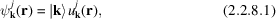where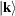denotes the plane wave (ignoring normalization) in Dirac's ket notation (2.2.5.3). The additional superscript j denotes the band index associated with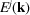(see Section 2.2.6.2). The two factors can be interpreted most easily for the two limiting cases, namely:

 (i) For a constant potential, for which the first factor corresponds to a plane wave with momentum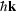[see (2.2.5.5)] but the second factor becomes a constant. Note that for a realistic (non-vanishing) potential, thevector of a Bloch function is no longer the momentum and thus is often denoted as pseudomomentum. (ii) If the atoms in a crystal are infinitely separated (i.e. for infinite lattice constants) the BZ collapses to a point, making the first factor a constant. In this case, the second factor must correspond to atomic orbitals and the label j denotes the atomic states 1s, 2s, 2p etc. In the intermediate case,is quantized [see (2.2.4.13)] and can take N values (or 2N states including spin) for N cells contained in the volume of the periodic boundary condition [see (2.2.4.21)]. Therefore, as the interatomic distance is reduced from infinity to the equilibrium separations, an atomic level j is broadened into a bandwith the quasi-continuousvectors and thus shows dispersion.

According to another theorem, the mean velocity of an electron in a Bloch state with wavevectorand energyis given by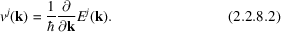If the energy is independent of, its derivative with respect tovanishes and thus the corresponding velocity. This situation corresponds to the genuinely isolated atomic levels (with band width zero) and electrons that are tied to individual atoms. If, however, there is any nonzero overlap in the atomic wavefunctions, thenwill not be constant throughout the zone.

In the general case, different notations are used to characterize band states. Sometimes it is more appropriate to label an energy band by the atomic level from which it originates, especially for narrow bands. In other cases (with a large band width) the free-electron behaviour may be dominant and thus the corresponding free-electron notation is more appropriate.